Area of combination of figures : circle based

Chapter 12 Class 10 Areas related to Circles
Concept wise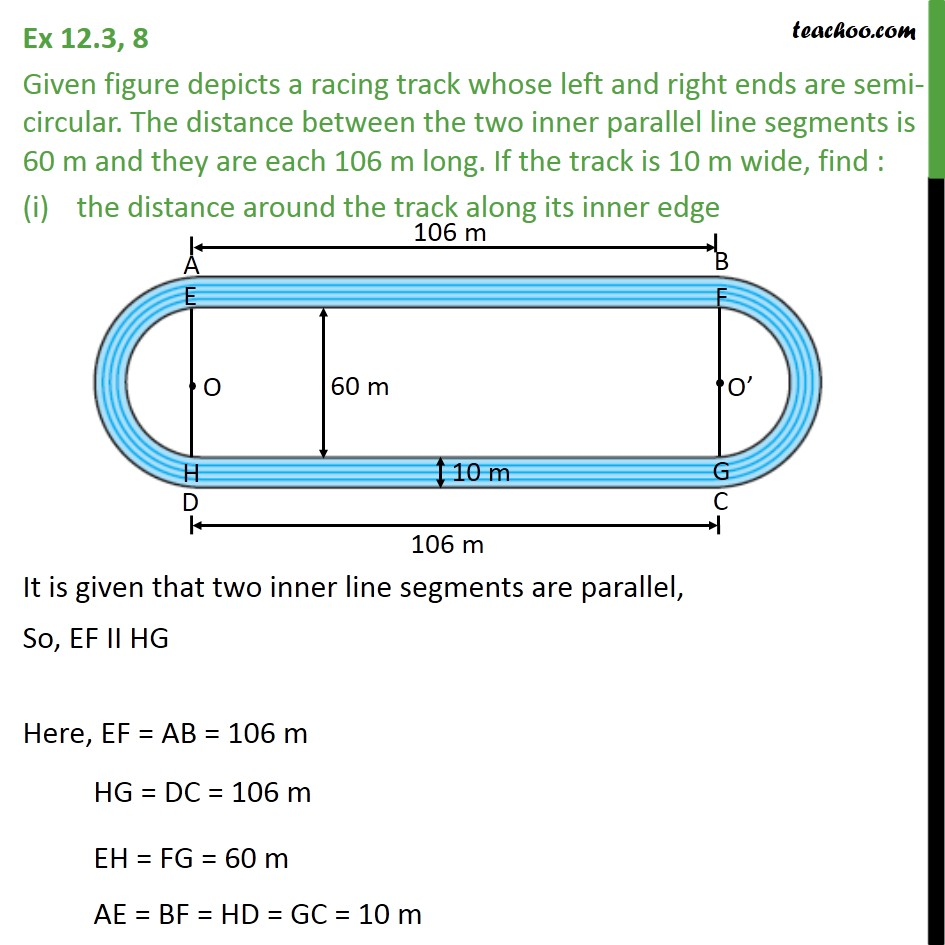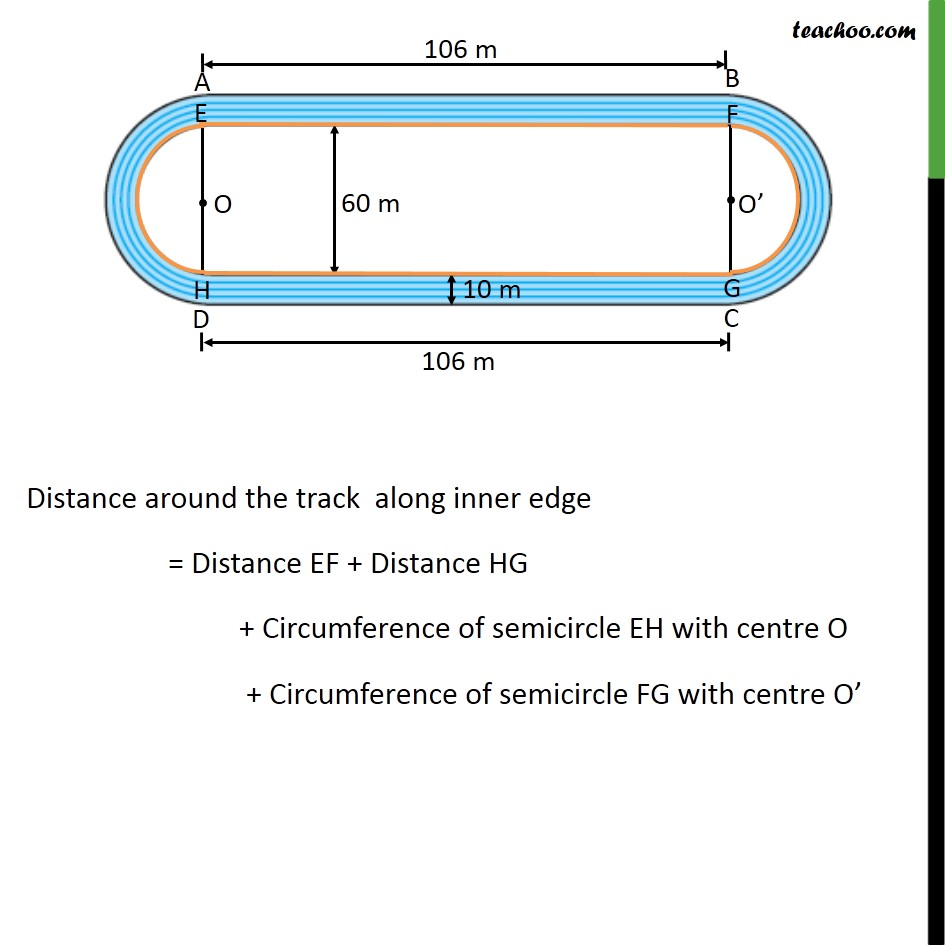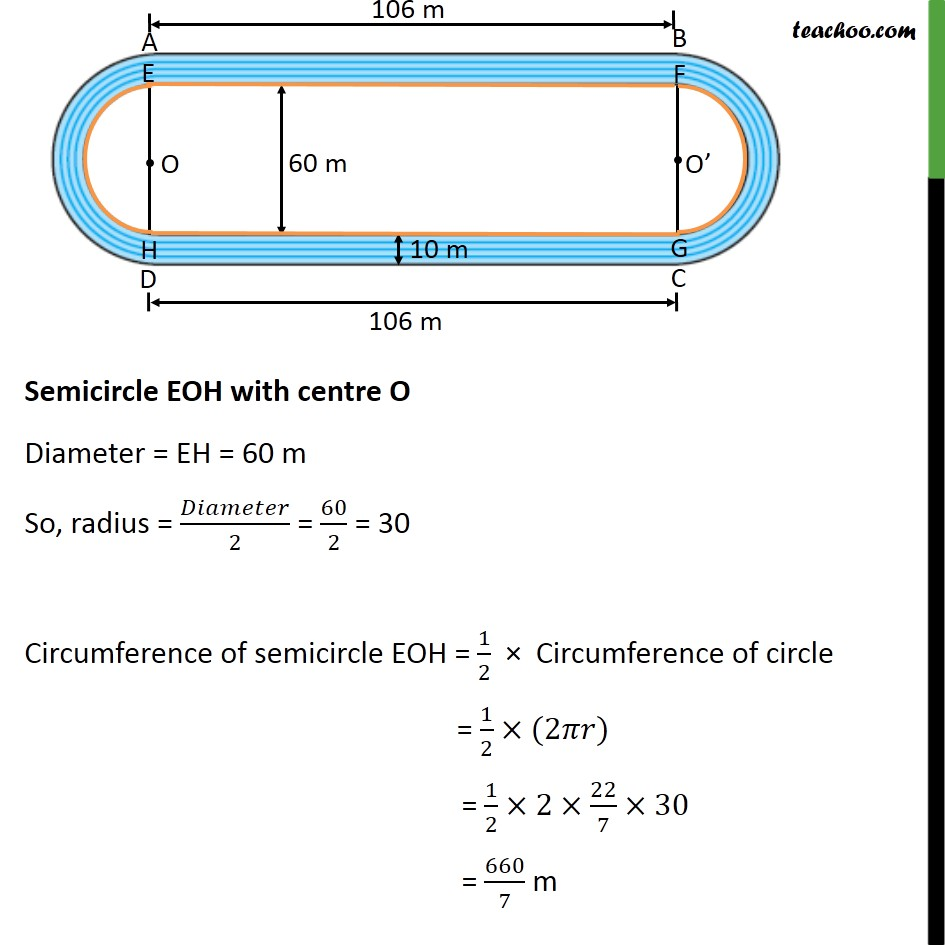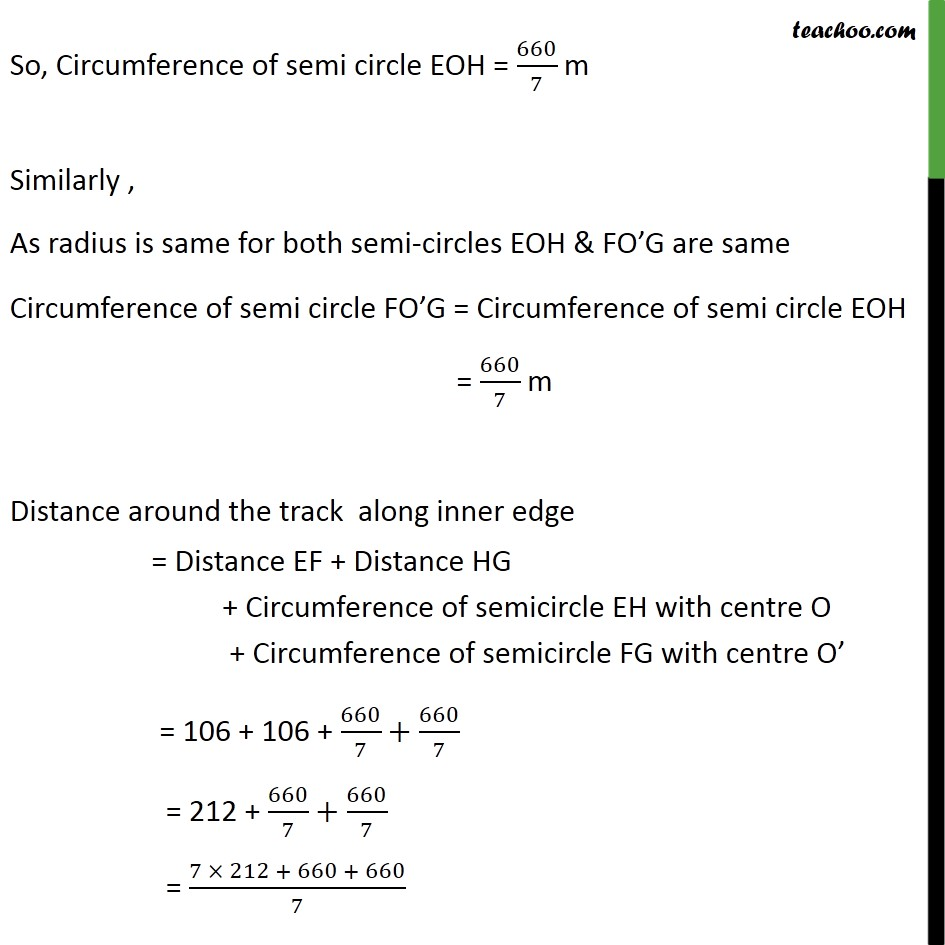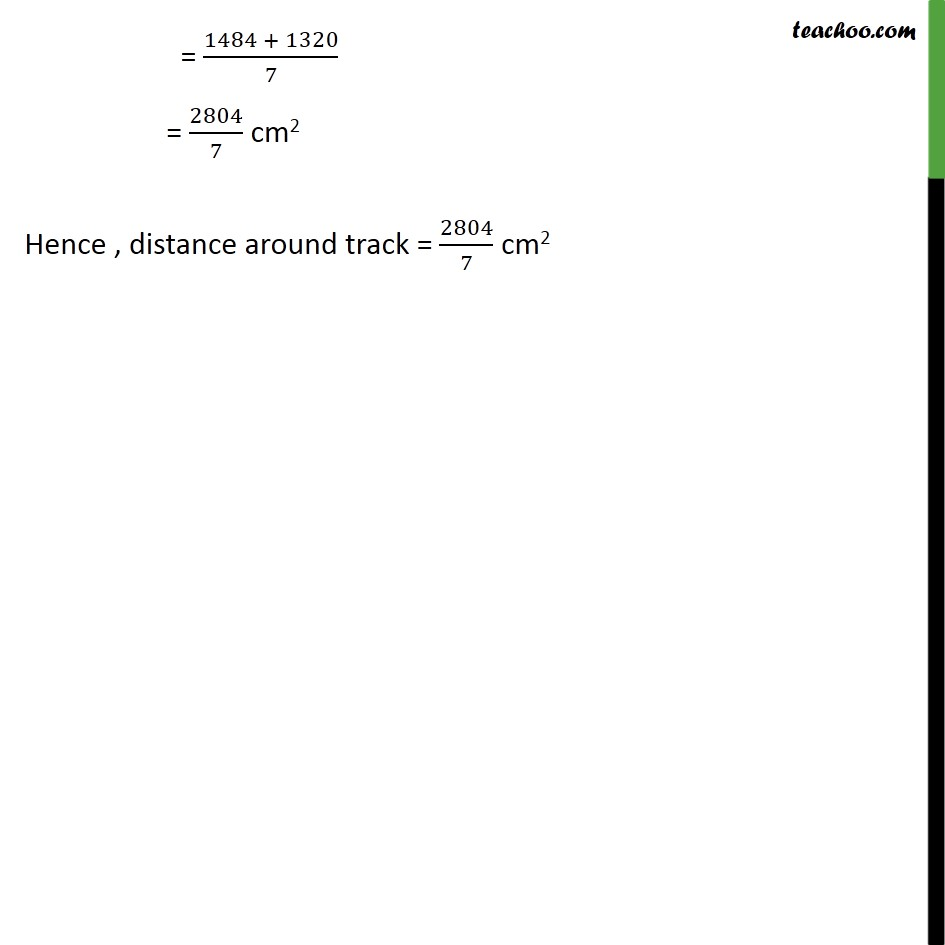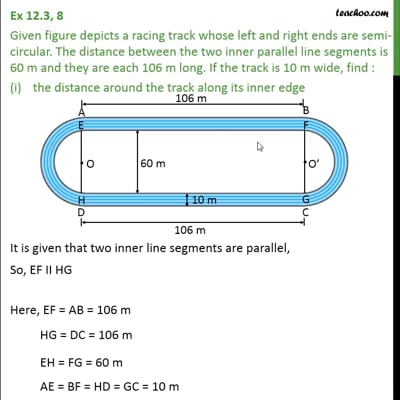This video is only available for Teachoo black users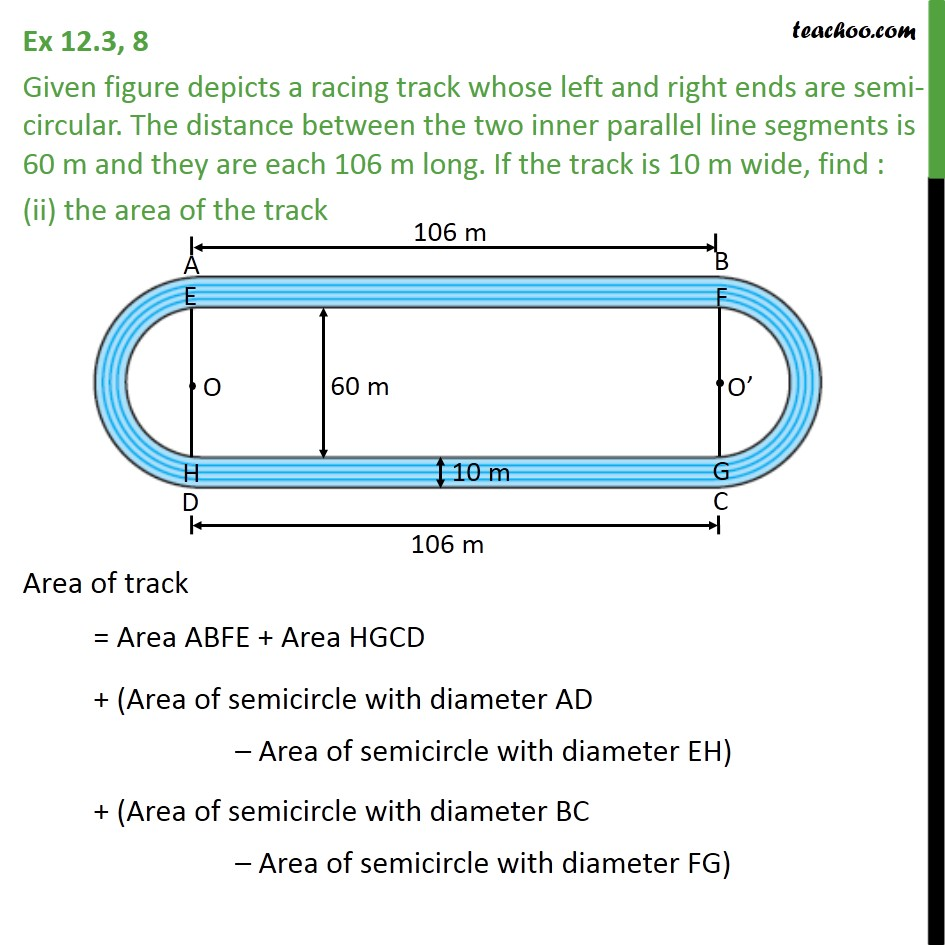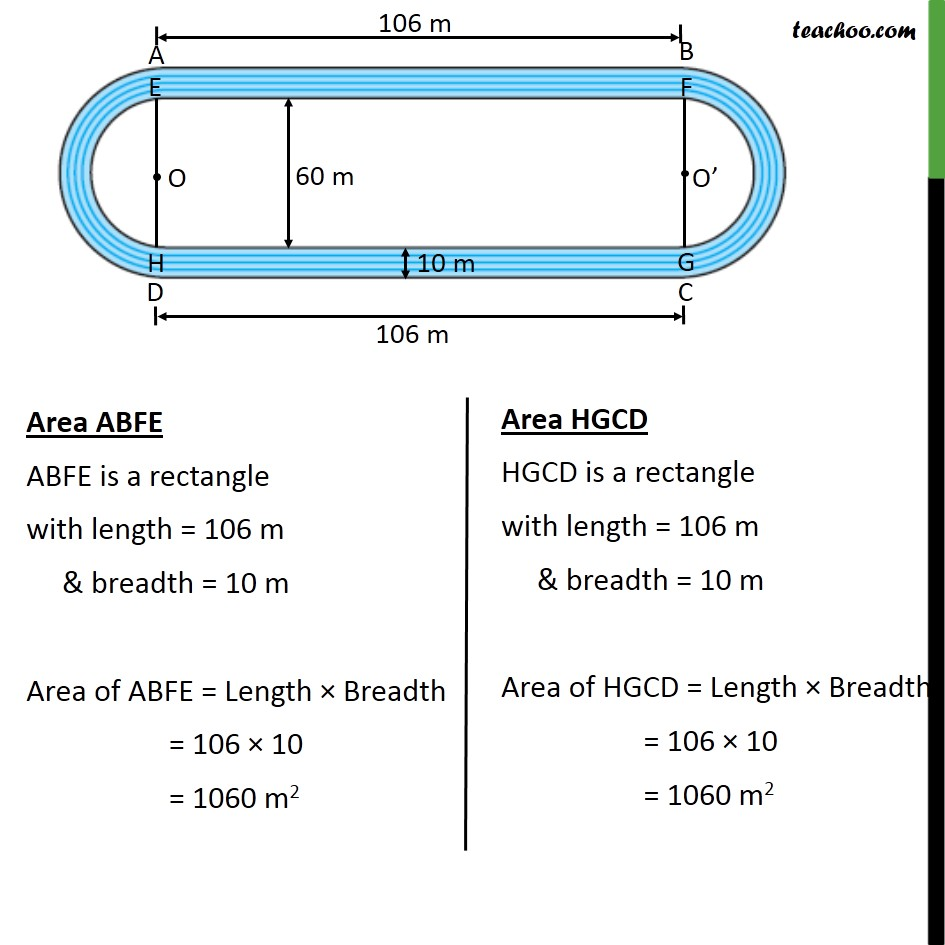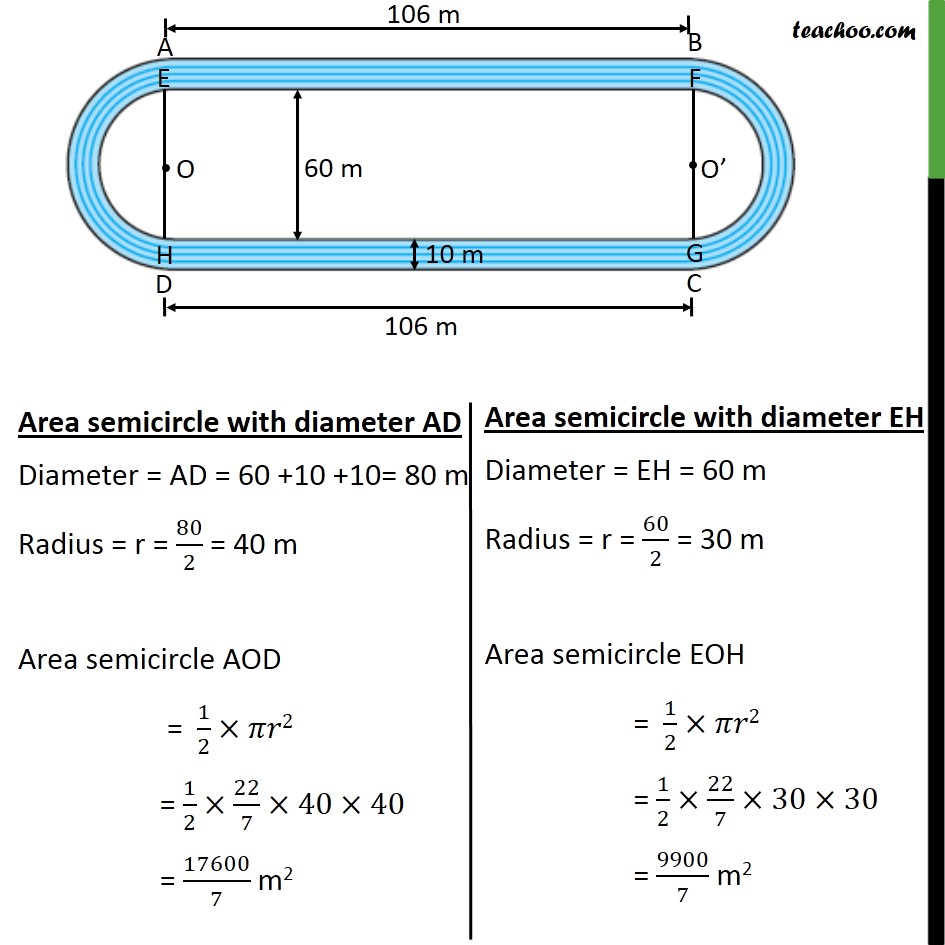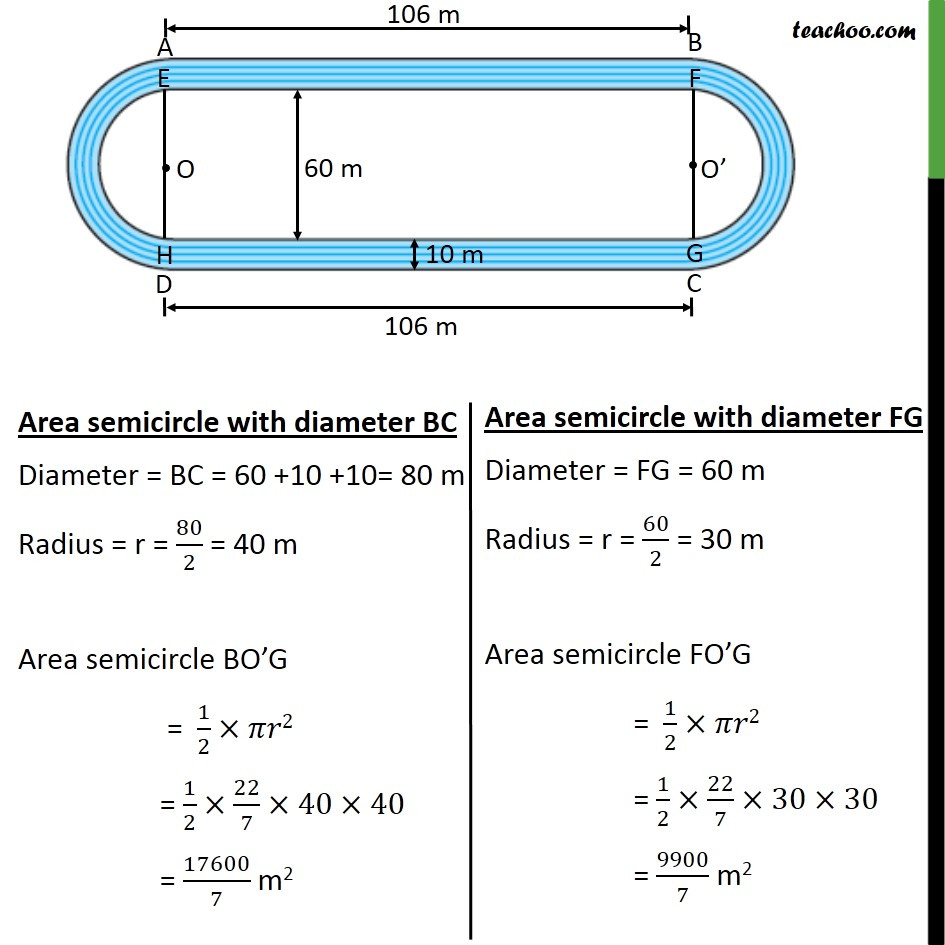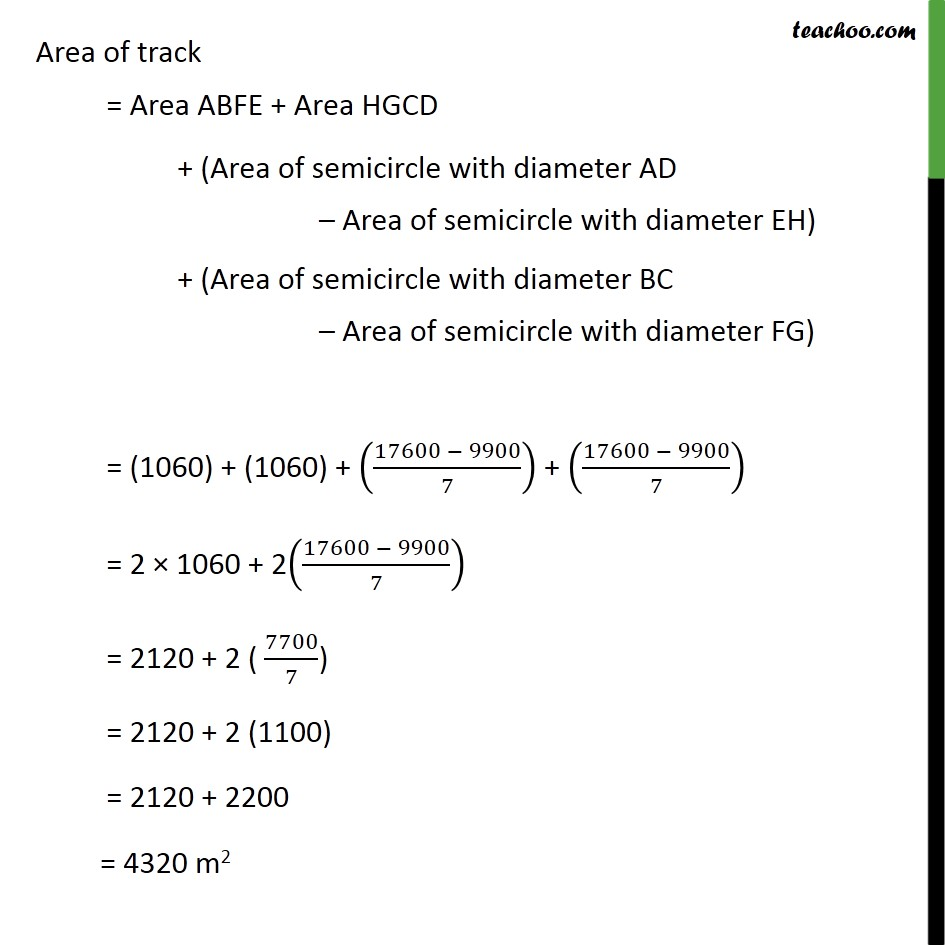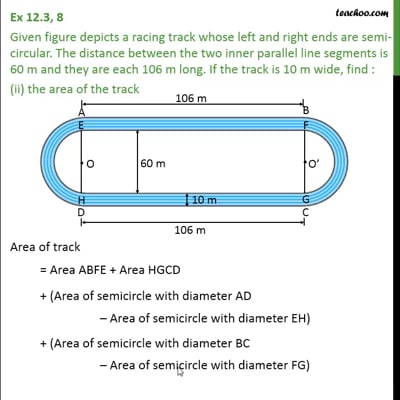This video is only available for Teachoo black users

Solve all your doubts with Teachoo Black (new monthly pack available now!)

### Transcript

Ex 12.3, 8 Given figure depicts a racing track whose left and right ends are semi-circular. The distance between the two inner parallel line segments is 60 m and they are each 106 m long. If the track is 10 m wide, find : the distance around the track along its inner edge It is given that two inner line segments are parallel, So, EF II HG Here, EF = AB = 106 m HG = DC = 106 m EH = FG = 60 m AE = BF = HD = GC = 10 m Distance around the track along inner edge = Distance EF + Distance HG + Circumference of semicircle EH with centre O + Circumference of semicircle FG with centre O Semicircle EOH with centre O Diameter = EH = 60 m So, radius = /2 = 60/2 = 30 Circumference of semicircle EOH = 1/2 Circumference of circle = 1/2 (2 ) = 1/2 2 22/7 30 = 660/7 m So, Circumference of semi circle EOH = 660/7 m Similarly , As radius is same for both semi-circles EOH & FO G are same Circumference of semi circle FO G = Circumference of semi circle EOH = 660/7 m Distance around the track along inner edge = Distance EF + Distance HG + Circumference of semicircle EH with centre O + Circumference of semicircle FG with centre O = 106 + 106 + 660/7+660/7 = 212 + 660/7+660/7 = (7 212 + 660 + 660)/7 = (1484 + 1320)/7 = 2804/7 cm2 Hence , distance around track = 2804/7 cm2 Ex 12.3, 8 Given figure depicts a racing track whose left and right ends are semi-circular. The distance between the two inner parallel line segments is 60 m and they are each 106 m long. If the track is 10 m wide, find : (ii) the area of the track Area of track = Area ABFE + Area HGCD + (Area of semicircle with diameter AD Area of semicircle with diameter EH) + (Area of semicircle with diameter BC Area of semicircle with diameter FG) Area ABFE ABFE is a rectangle with length = 106 m & breadth = 10 m Area of ABFE = Length Breadth = 106 10 = 1060 m2 Area of track = Area ABFE + Area HGCD + (Area of semicircle with diameter AD Area of semicircle with diameter EH) + (Area of semicircle with diameter BC Area of semicircle with diameter FG) = (1060) + (1060) + ((17600 9900)/7) + ((17600 9900)/7) = 2 1060 + 2((17600 9900)/7) = 2120 + 2 ( 7700/7) = 2120 + 2 (1100) = 2120 + 2200 = 4320 m2